Tangram
 To my Main Page    "Mathematische Basteleien"
What is Tangram?
Tangram is one of the most popular games to lay.
You put figures of 7 pieces together (five triangles, one square and one parallelogram). You must use all pieces. They must touch but not overlap.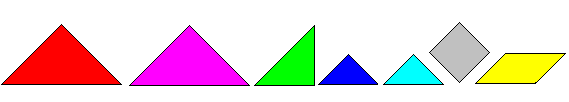Main Problem  top
 All seven tangram pieces consist of half squares with this shape: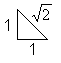.
There are 32 half squares or 16 squares altogether.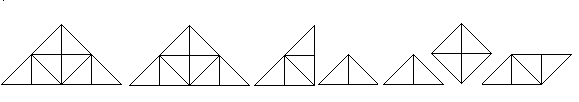...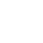... You can build a 4x4-square with all the 16 squares. The main problem of the 'tangram research' is building a square with all the 7 pieces.

You can also choose the smallest tangram piece, the blue triangle, as the basic triangle. I took the half triangle as the basic form, because the square built of all seven tangram pieces has the simple length 4.
 Basic triangle on this page:............................... Another way: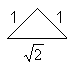................
The difference is: You have to change the rational und irrational length of a side.

Building Figures
1st problem: New figures     top
 ...... You can invent new figures. A figure is good, if you recognize it by seeing.There are thousands of figures, which people have already built.
 ...... [3sqrt(2)]x[3sqrt(2)]-squares are possible, if you leave blank one triangle or two.2nd problem: Filling given shapes top
 ......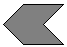..... You must find the right position of the tangram pieces filling this shape.  (Solution in the end of this chapter)

3rd problem: How many possibilities are there to lay the same figure?    top
 ...... You can lay the trapezium in two different ways....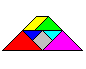... This trapezium is not a solution. If you lay this figure, you find the mistake:  The yellow and the green piece are a little bit bigger. You use the fact, that 4 and the triple of the square root of 2 (=4.24) are about the same.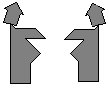Other paradoxes comparing two similar tangram figures, which seem to be alike. An example by H.Dudeney Solution: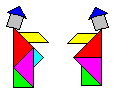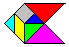Tangram Birds  top
About 100 students (aged 11/12/13) got the task to design birds by tangram pieces.
Here is a small selection of nice birds.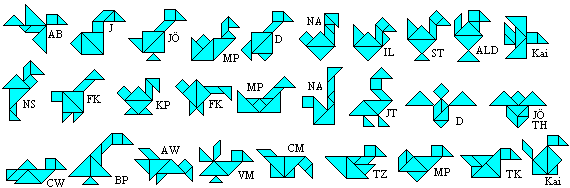Not all the students became friends with tangram pieces:[= I hate tangrams ;-).]
(Thanks to 6b, 6c, 7a, 7c, 7d in 1999/2000)

Paul's Men
My grandson Paul (11) sent me the following figures for this page.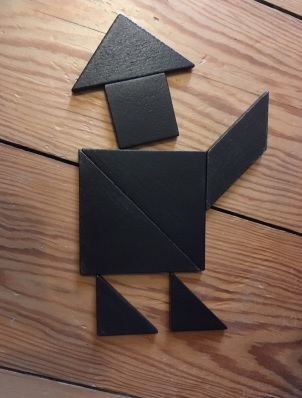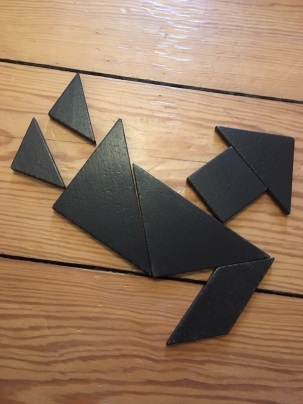Classifying Figures top
You can follow the positions of the sides of the half squares.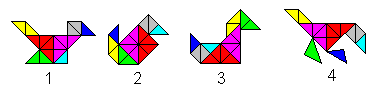1      the sides at the right angle are horizontal or vertical
2      the side opposite the right angle is horizontal or vertical
3      mixture of 1 and 2
4      any position

If you have a mathematical point of view, you must allow only 1 and 2.
Nearly all tangram figures belong to model 4. There are many nice and expressive forms, because there are less rules. They are organized by topics.

Convex Figures  top
'A figure is convex', means: If you choose any two points inside the figure, the whole line between the points must also be inside the figure.Surprising: There are only 13 convex figures, you can build from tangram pieces.
Proof by Fu Traing Wang and Chuan-Chih Hsiung in 1942 (Book 4)

Grid Tangrams with Convex Perimeter     top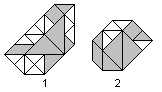You find an interesting suggestion in book 3 and book 4 to classify tangram figures. This only refers to 'mathematical' figures', which bird 1 and bird 2 (see above) stand for. You can lay them into a coordinate system, so that the corners of the seven tangram pieces have integers as coordinates. In other words: You can order the tangram pieces in a way that the sides with the unit 1 are horizontal or vertical. The lines with the unit (root of 2) are diagonal.
The figures are widened by little (white) triangles as necessary, so that a convex figure develops. These triangles have the same size as the blue tangram pieces. You count the triangles. Bird 1 needs 14 triangles and is 14-convex. Bird 2 is 5-convex. The convex figures don't need a triangle. They are 0-convex. You find all 133 (abstract) 1-convex tangram figures and solutions in book 4.

There is the problem to find figures with the largest convex perimeter.
Bruno Curfs found the following seven 41-convex tangrams. Probably 41 is the upper limit (5).
...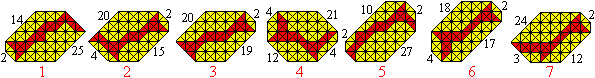...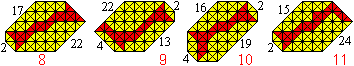... I received more 41-convex tangrams: 8 from Ludwig Welther, 9 from Hartmut Blessing,  10 and 11 from Hannes Georg Kuchler.

 ...... Daniel Gronau checked all possible grid tangrams by his computer. He found out that there are three more solutions.

Bruno Curfs proved mathematically that 44-konvex is an upper limit (5).

Making of Tangram Pieces top
Probably the tangram pieces are developed from cutting a 4x4-square in pieces.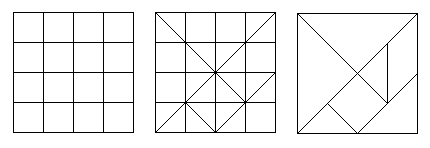You use it to make tangram pieces. You draw a 4x4-square with some diagonals on plywood or on cardboard. Then you saw or cut the pieces as shown at the drawing.

ALOIS STUDER MADE BOBBIN LACES OF THE SEVEN TANGRAM PIECES.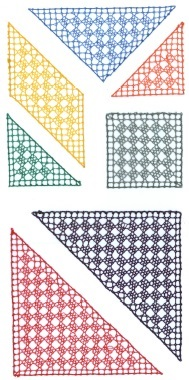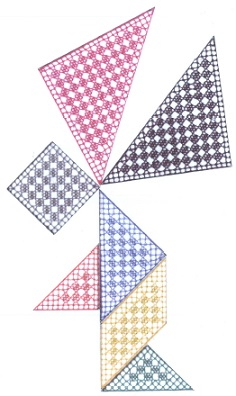Angel

Now (July 8th) Alois Studer sent me another artistic work.
It is very interesting, because he formed a square by two big, three small and two very small tangram sets.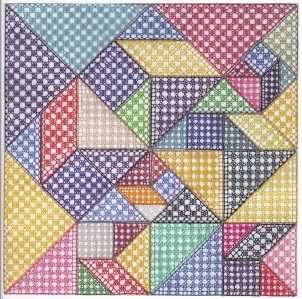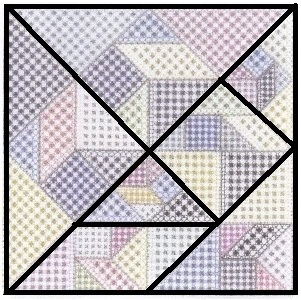Variants of the Tangram Game     top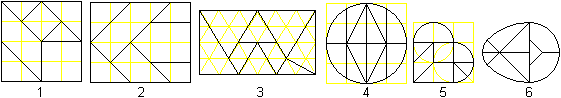You can make more tangram games, if you divide simple geometric figures like square, rectangle or circle. The most famous are (1) "Pythagoras",  (2) "Kreuzbecher",  (3) "Alle Neune",  (4) "Circular Puzzle",  (5) "The Broken Heart", and (6) "The Magic Egg".
Here is a wide field for designing your own tangram pieces and playing with them.

Tangram on the Internet top

German

Claus Michael Ringel
Tangram

Gerd Müller
Tangram interaktiv
 Hartmut Blessing DER IQ-BLOCK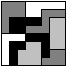Herbert Hertramph
Tangram-Spiel von Jos van Uden, Tangram-Spiel von Serj Dolgav zum Herunterladen

Michael Bischoff
Tangram for you

stopkidsmagazin
Tangram online

tan-gram
tangram mit einer galerie von 75 exponaten

Wikipedia
Tangram

English

Andrew D. Orlov
Tangram House

Barbara E. Ford
Tangrams - The Magnificent Seven Piece Puzzle

Gianni A. Sarcone and Marie-Jo Waeber
Tangram, the incredible timeless 'Chinese' puzzle
 Hartmut Blessing IQ-BLOCKMarie-Jo Waeber and Gianni A. Sarcone   (Archimedes' Laboratory)
Tangramagic

Michael Bischoff
Tangram for you

Paul Scott
CONVEX TANGRAMS

Wikipedia
Tangram

References  (German) top
(1) Pieter van Delft, Jack Botermans: Denkspiele der Welt, München 1998
(2) Karl-Heinz Koch: ...lege Spiele, Köln 1987 (dumont taschenbuch1480)
(3) Rüdiger Thiele, Konrad Haase: Teufelsspiele, Leipzig 1991
(4) Joost Elffers, Michael Schuyt: Tangram, Dumont, Köln 1997 (+ tangram pieces)
(5) Bruno Curfs: Mathematical Tangram, CFF, newsletter of the "Nederlandse Kubus Club" NKC, 65 (November 2004)
(6) Jerry Slocum, Dieter Gebhardt, Jack Botermans, Monica Ma, Xiaohe Ma: The Tangram Book, 2003
[ISBN 1-4027-0413-5] Sterling Publishing Company

Feedback: Email address on my main page